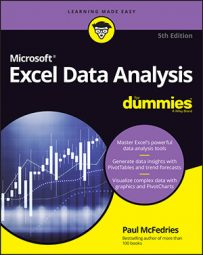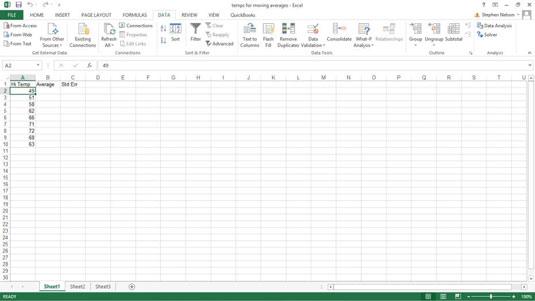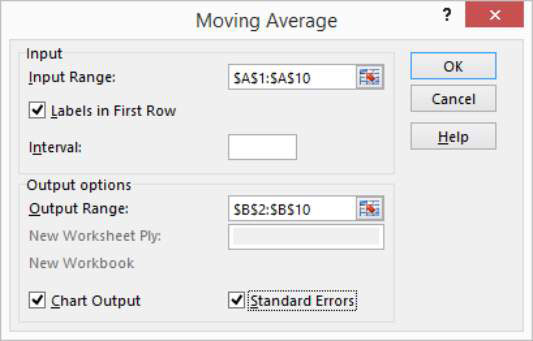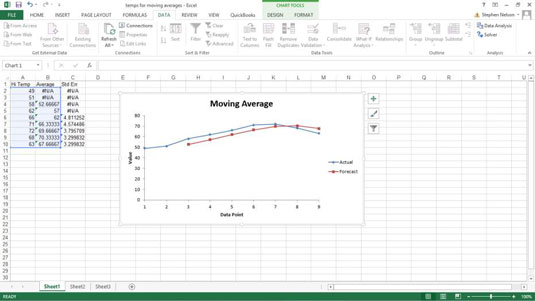##### Excel Data Analysis For DummiesThe Data Analysis command provides a tool for calculating moving and exponentially smoothed averages in Excel. Suppose, for sake of illustration, that you’ve collected daily temperature information. You want to calculate the three-day moving average — the average of the last three days — as part of some simple weather forecasting. To calculate moving averages for this data set, take the following steps.1. To calculate a moving average, first click the Data tab’s Data Analysis command button.

2. When Excel displays the Data Analysis dialog box, select the Moving Average item from the list and then click OK.

Excel displays the Moving Average dialog box.3. Identify the data that you want to use to calculate the moving average.

Click in the Input Range text box of the Moving Average dialog box. Then identify the input range, either by typing a worksheet range address or by using the mouse to select the worksheet range.

Your range reference should use absolute cell addresses. An absolute cell address precedes the column letter and row number with \$ signs, as in \$A\$1:\$A\$10.

If the first cell in your input range includes a text label to identify or describe your data, select the Labels in First Row check box.

4. In the Interval text box, tell Excel how many values to include in the moving average calculation.

You can calculate a moving average using any number of values. By default, Excel uses the most recent three values to calculate the moving average. To specify that some other number of values be used to calculate the moving average, enter that value into the Interval text box.

5. Tell Excel where to place the moving average data.

Use the Output Range text box to identify the worksheet range into which you want to place the moving average data. In the worksheet example, the moving average data has been placed into the worksheet range B2:B10.

6. (Optional) Specify whether you want a chart.

If you want a chart that plots the moving average information, select the Chart Output check box.

7. (Optional) Indicate whether you want standard error information calculated.

If you want to calculate standard errors for the data, select the Standard Errors check box. Excel places standard error values next to the moving average values. (The standard error information goes into C2:C10.)

8. After you finish specifying what moving average information you want calculated and where you want it placed, click OK.

Excel calculates moving average information.Note: If Excel doesn't have enough information to calculate a moving average for a standard error, it places the error message into the cell. You can see several cells that show this error message as a value.